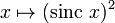# Sinc-squared function

(diff) ← Older revision | Latest revision (diff) | Newer revision → (diff)
Jump to: navigation, search
This article is about a particular function from a subset of the real numbers to the real numbers. Information about the function, including its domain, range, and key data relating to graphing, differentiation, and integration, is presented in the article.
View a complete list of particular functions on this wiki
For functions involving angles (trigonometric functions, inverse trigonometric functions, etc.) we follow the convention that all angles are measured in radians. Thus, for instance, the angle of$90\,^\circ$ is measured as$\pi/2$.

## Definition

This function is defined as the composite of the square function and the sinc function. Explicitly, it is given as:$x \mapsto (\operatorname{sinc} \ x)^2$

Alternatively, it is given as:

$array}{rl} 1, & x = 0 \\ \frac{\sin^2x}{x^2}, & x \ne 0 \\\end{array$

## Graph

Here is a graph on the interval$[-3\pi,3\pi]$:

The graph is a little unclear, here is an alternative version where different scalings are used for the$x$-axis and$y$-axis: